Author: Oscar Cronquist Article last updated on August 15, 2022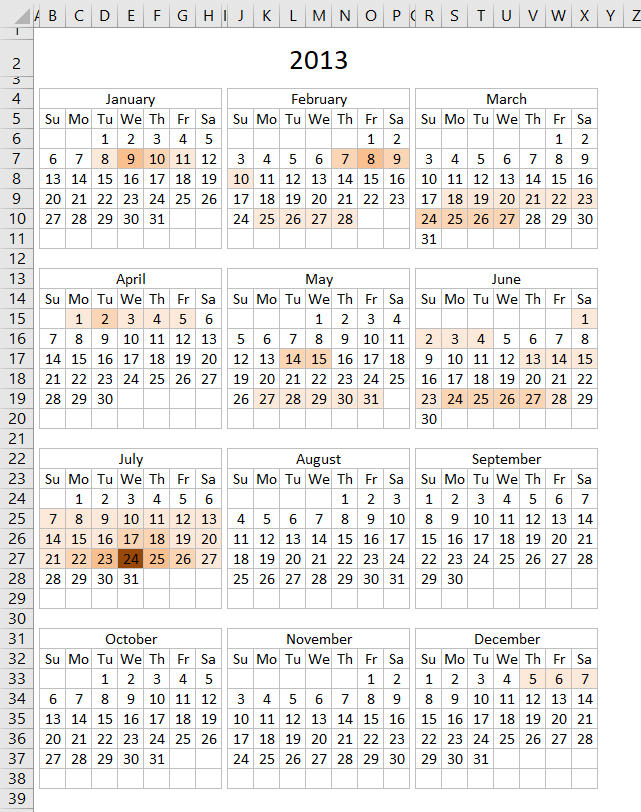The calendar shown in the image above highlights events based on frequency. It is made only with a few conditional formatting formulas and an Excel defined table that allows you to add new events or edit/delete old ones.

Conditional formatting + Excel Table

Macro + Excel Table

## 1. Conditional formatting formulas

What you perhaps want to customize is how events are highlighted. The following table shows how conditional formatting is used.

 Event frequency CF formula 1 =SUMPRODUCT((INT(INDIRECT("Table1[Start]"))<=B6)* (INT(INDIRECT("Table1[End]"))>=B6))=1 2 =SUMPRODUCT((INT(INDIRECT("Table1[Start]"))<=B6)* (INT(INDIRECT("Table1[End]"))>=B6))=2 3 =SUMPRODUCT((INT(INDIRECT("Table1[Start]"))<=B6)* (INT(INDIRECT("Table1[End]"))>=B6))=3 4 =SUMPRODUCT((INT(INDIRECT("Table1[Start]"))<=B6)* (INT(INDIRECT("Table1[End]"))>=B6))=4

This image shows the rules manager for conditional formatting formulas, it shows you the fill color I used and the calendar cell range it is applied to.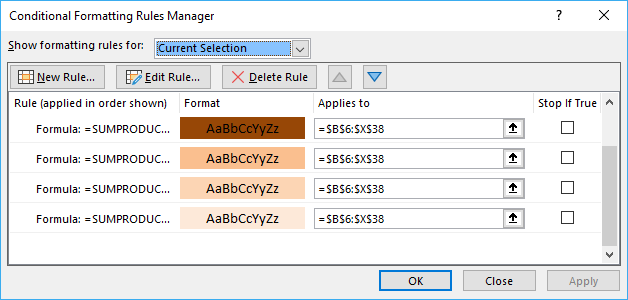## 2. Explaining CF formula

The following CF formula is the one that identifies dates that have only one event scheduled.

=SUMPRODUCT((INT(INDIRECT("Table1[Start]"))<=B6)* (INT(INDIRECT("Table1[End]"))>=B6))=1

#### Step 1 - Check date in calendar with start column in Excel table

The INDIRECT function returns the cell reference based on a text string and shows the content of that cell reference.

Function syntax: INDIRECT(ref_text, [a1])

Check if date in cell B6 is larger than or equal to start dates. The INDIRECT function is needed to be able to use an Excel table name in a Conditional Formatting formula.

The INT function removes the decimal part from positive numbers and returns the whole number (integer) except negative values are rounded down to the nearest integer.

Function syntax: INT(number)

INT(INDIRECT("Table1[Start]"))<=B6

becomes

INT({41282.375; 41330.3333333333; 41312.3333333333; 41351.4166666667; 41365.5; 41421.75; 41438.3333333333; 41448.625; 41283.3333333333; 41283.3333333333; 41312.3333333333; 41313.3333333333; 41314.3333333333; 41357.4166666667; 41366.5; 41408.75; 41408.75; 41449.75; 41462.75; 41472.75; 41613; 41477; 41478; 41479})<=B6

becomes

{41282; 41330; 41312; 41351; 41365; 41421; 41438; 41448; 41283; 41283; 41312; 41313; 41314; 41357; 41366; 41408; 41408; 41449; 41462; 41472; 41613; 41477; 41478; 41479}<=41273

and returns

{FALSE; FALSE; FALSE; FALSE; FALSE; FALSE; FALSE; FALSE; FALSE; FALSE; FALSE; FALSE; FALSE; FALSE; FALSE; FALSE; FALSE; FALSE; FALSE; FALSE; FALSE; FALSE; FALSE; FALSE}

#### Step 2 - Check date in calendar with end column in Excel table

INT(INDIRECT("Table1[End]"))>=B6))

returns

{TRUE; TRUE; TRUE; TRUE; TRUE; TRUE; TRUE; TRUE; TRUE; TRUE; TRUE; TRUE; TRUE; TRUE; TRUE; TRUE; TRUE; TRUE; TRUE; TRUE; TRUE; TRUE; TRUE; TRUE}

#### Step 3 - Multiply conditions (AND logic)

In order to identify a date inside an event range both conditions must be met.

(INT(INDIRECT("Table1[Start]"))<=B6)* (INT(INDIRECT("Table1[End]"))>=B6)

becomes

{FALSE; FALSE; FALSE; FALSE; FALSE; FALSE; FALSE; FALSE; FALSE; FALSE; FALSE; FALSE; FALSE; FALSE; FALSE; FALSE; FALSE; FALSE; FALSE; FALSE; FALSE; FALSE; FALSE; FALSE} * {TRUE; TRUE; TRUE; TRUE; TRUE; TRUE; TRUE; TRUE; TRUE; TRUE; TRUE; TRUE; TRUE; TRUE; TRUE; TRUE; TRUE; TRUE; TRUE; TRUE; TRUE; TRUE; TRUE; TRUE}

and returns

{0; 0; 0; 0; 0; 0; 0; 0; 0; 0; 0; 0; 0; 0; 0; 0; 0; 0; 0; 0; 0; 0; 0; 0}.

#### Step 4 - Sum events

Function syntax:

SUMPRODUCT((INT(INDIRECT("Table1[Start]"))<=B6)* (INT(INDIRECT("Table1[End]"))>=B6))

becomes

SUMPRODUCT({0; 0; 0; 0; 0; 0; 0; 0; 0; 0; 0; 0; 0; 0; 0; 0; 0; 0; 0; 0; 0; 0; 0; 0})

and returns 0 (zero).

#### Step 5 - Check if the number of events equal 1

If the sum is equal to 1 then the Conditional formatting formula returns TRUE and the cell is highlighted, if not then the formula returns FALSE and the cell is not highlighted.

SUMPRODUCT((INT(INDIRECT("Table1[Start]"))<=B6)* (INT(INDIRECT("Table1[End]"))>=B6))=1

becomes

0=1

and returns FALSE. Cell B6 is not highlighted.

## 3. Populating the Excel table

The following picture shows the Excel defined table containing the data about each event, the event name, when it starts and when it ends.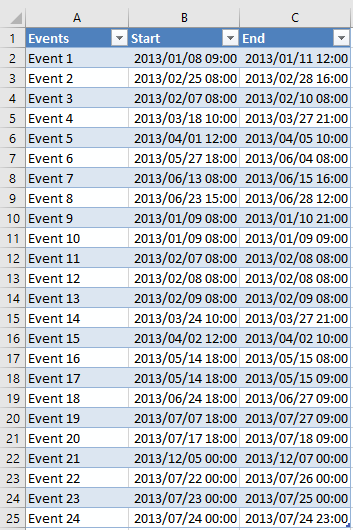## 4. CF formula examples

If you want to highlight cells that have less then 5 events scheduled with a given color then use this CF formula:

=SUMPRODUCT((INT(INDIRECT("Table1[Start]"))<=B6)* (INT(INDIRECT("Table1[End]"))>=B6))<5

If you want to highlight cells that have greater then 5 events and less than 10 events scheduled with a given color then use this CF formula:

=(SUMPRODUCT((INT(INDIRECT("Table1[Start]"))<=B6)* (INT(INDIRECT("Table1[End]"))>=B6))<10)* (SUMPRODUCT((INT(INDIRECT("Table1[Start]"))<=B6)* (INT(INDIRECT("Table1[End]"))>=B6))>5)

There are also CF formulas that hide dates and formatting on each month because some cells show the previous or the next months dates. The calendar looks cleaner without them.

## 5. Get Excel *.xlsx

Heat map calendar.xlsx

Note that no VBA is used in this workbook, however, there is a VBA solution demonstrated below if you prefer that.

## 6. Heat map - VBA Solution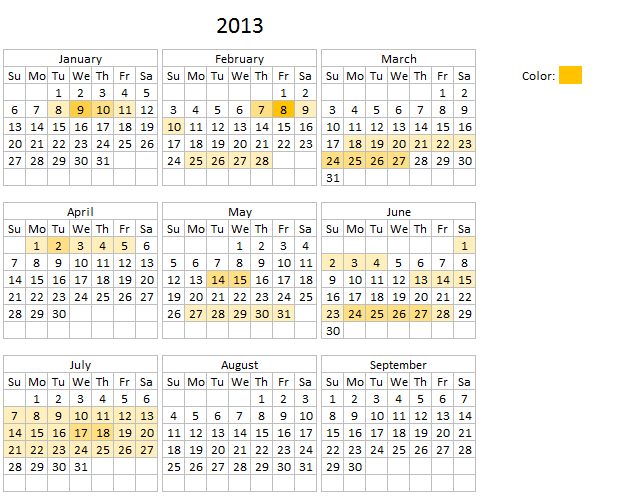Hi, I would like to use this example with my dataset, however, I'd like to visually show the number of events per date to understand when are we the busiest, slowest, etc. and be able to forecast using this data.

Ideally, I would like some sort of data bar or color change indicating the level for each date (Jan 1 has 10 items while Jan 2 has 3 and I can visually see that in each cell instead of seeing numbers or a solid color for each cell (here yellow and blue).

This article demonstrates how to highlight events on a yearly calendar based on frequency per day. You will find a link to this workbook at the end of this article.

The color on the calendar gives a rough estimate on the number of events per date.

• No color no events.
• Light color one or a few events.
• Darker color means many events.

You add, edit or delete events to worksheet "Table" and every time you go back to worksheet "Calendar" the colors are refreshed by the macro below.

There is a specific cell next to the calendar that allows you to change the highlight color if you prefer. Press with mouse on that cell and change the cell color to a color you want.

## 7. VBA code

1. Press with right mouse button on on sheet Calendar
2. Press with left mouse button on "View Code"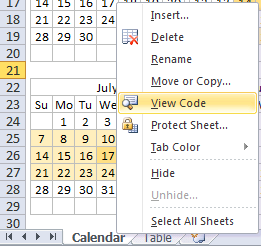3. Copy vba code below
4. Paste code to sheet module
5. Exit VB Editor
```Private Sub Worksheet_Activate()
Dim CRng As Variant
Dim Dt As Variant
Dim CDt As Variant
Dim Cnt As Integer
Dim r As Long
Dim c As Long
Dim St As Integer
Application.ScreenUpdating = False
CRng = Worksheets("Calendar").Range("B6:X38").Value
With Worksheets("Table")
For r = 1 To UBound(CRng, 1)
For c = 1 To UBound(CRng, 2)
If CRng(r, c) <> "" Then
For CDt = 1 To .Range("Table1[Start]").Cells.Count
If CRng(r, c) >= Int(.Range("Table1[Start]").Cells(CDt).Value) And CRng(r, c) <= Int(.Range("Table1[End]").Cells(CDt).Value) Then Cnt = Cnt + 1 End If Next CDt End If If Cnt > St Then St = Cnt
Cnt = 0
Next c
Next r
End With
Set Rng = Worksheets("Calendar").Range("B6:X38")
'Remove previous formatting
With Rng.Interior
.Pattern = xlNone
End With
With Worksheets("Table")
For Each Dt In Worksheets("Calendar").Range("B6:X38")
For CDt = 1 To .Range("Table1[Start]").Cells.Count
If Dt >= Int(.Range("Table1[Start]").Cells(CDt).Value) And Dt <= Int(.Range("Table1[End]").Cells(CDt).Value) Then Cnt = Cnt + 1 End If Next CDt If Cnt > 0 Then
With Dt.Interior
.Pattern = xlSolid
.PatternColorIndex = xlAutomatic
.Color = Worksheets("Calendar").Range("AB5").Interior.Color ' xlThemeColorAccent4 '
.TintAndShade = 1 - (Cnt / St)
End With
'Reset counter a
Cnt = 0
End If
Next Dt
End With
Application.ScreenUpdating = True
End Sub
```

### WorkSheet "Table"

The picture below shows the events in an Excel defined table named [Table1].

You don't need to adjust cell references or formulas everything is automatic, Excel defined Tables are great in that aspect.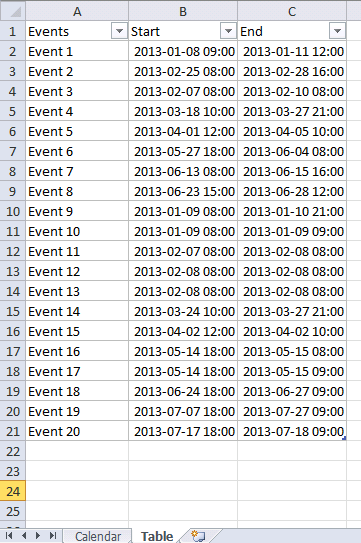You can find a heat map monthly calendar here.

## 8. Get excel *.xlsm file

### Get the Excel fileHeat-map-calendar.xlsm